Chapter 18. Chemical Thermodynamics

# End-of-Chapter Material

Jessie A. Key

Exercises

1. Classify each of the following as spontaneous or nonspontaneous processes:
1. the browning of a cut apple slice on a snack tray over time
2. the rolling of a ball uphill
3. the formation of diamond from the graphite in your pencil
4. the melting of ice cubes in a glass of water you are holding
2. State the second law of thermodynamics.
3. What sign would you expect for ΔS for the following processes?
1. water freezing in a lake during a cold Alberta winter
2. AB(s) + CD(s) → AC(g) + BD(g)
3. a balloon with a fixed amount of gas stretched to a larger volume
4. the sublimation of dry ice
5. 3E2F2(g) → E6F6(g)
4. Which of the following would you expect to have the higher standard molar entropy, S°?
1. Br2() or Br2(g)
2. NO2(g) or NO(g)
3. C2H6(g) or C5H12(g)
5. Calculate the ΔS° of the following reactions using the values listed in the appendix.
1. 4NH3(g) + 5O2(g) → 6H2O(g)  + 4NO(g)
2. 2HgO(s) → 2Hg()  + O2(g)
3. 3FeCl2(s) + KNO3(s) + 4HCl (aq) → 3FeCl3(s) + KCl(s) + NO(g) + 2H2O()
6. Draw a diagram showing the approximate entropy change of water from −100°C to 250°C.
7. A chemical reaction has ΔH° = −43.5 kJ and ΔS° = −65.8 J/K. Calculate ΔG° at 298 K. Is this a spontaneous process?
8. Using data from the appendix, determine ΔG° for all reactions listed in question 5.
9. Find the standard Gibbs energy change for the reaction CaCO3(s) → CaO(s) + CO2(g).

The ΔGf° values for the three components of this reaction system are CaCO3(s): –1128 kJ mol–1; CaO(s): –603.5 kJ/mol; CO2(g): –137.2 kJ/mol.

10. Using the appendix data, determine the approximate temperature at which the following processes are at equilibrium:
1. CH2Cl2() → CH2Cl2(g)
2. CH3OH() → CH3OH(g)
11. Determine if the following reactions would be spontaneous at any temperature, nonspontaneous at any temperature, spontaneous at low temperature but not high temperature, or spontaneous at high temperature but not low temperature:
1. ΔH° = −750 kJ and ΔS° = 250 J/K
2. ΔH° = −100 kJ and ΔS° = −300 J/K
3. ΔH° = 95 kJ and ΔS° = −70 J/K
4. ΔH° = 400 kJ and ΔS° = 500 J/K
12. The Ka for acetic acid, CH3COOH, is 1.75 × 10−5 at 25°C.
1. Using the Ka value, determine the ΔG° for the dissociation of acetic acid in aqueous solution.
2. What is the value of ΔG when [H+] is 7.5 × 10−2 M, [CH3COO] is 2.5 × 10−2 M, and [CH3COOH] is 0.10 M?
13. Calculate the equilibrium constant for the reaction H+(aq) + OH(aq) → H2O() from the following data:
 H+(aq) OH−(aq) H2(ℓ) ΔH°f, kJ mol–1 0 –230.0 –285.8 S°, J K–1 mol–1 0* –10.9 70.0

*Note that the standard entropy of the hydrogen ion is zero by definition. This reflects the fact that it is impossible to carry out thermodynamic studies on a single-charged species. All ionic entropies are relative to that of H+(aq), which explains why some values (as for the aqueous hydroxide ion) are negative.

14. Using data from the appendix, calculate the equilibrium constants at 298 K for all the reactions in question 5.

1. spontaneous
2. nonspontaneous
3. nonspontaneous
4. spontaneous
1. ΔS = −
2. ΔS = +
3. ΔS = +
4. ΔS = +
5. ΔS = −
1.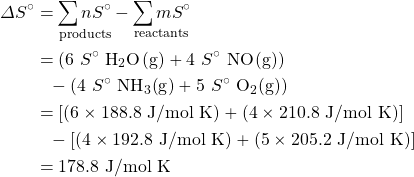2.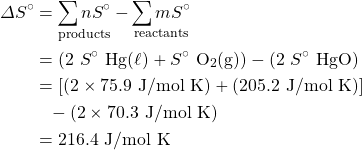3.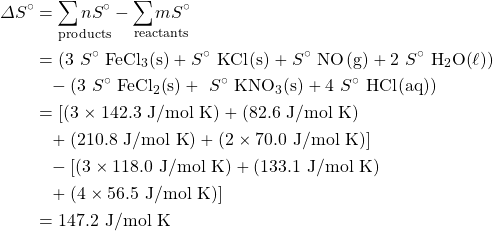1.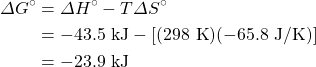ΔG° is negative. Therefore, this is a spontaneous process.

1. ΔG° = (–603.5 – 137.2) – (–1128) kJ/mol = +130.9 kJ/mol, indicating that the process is not spontaneous under standard conditions (i.e., solid calcium carbonate will not form solid calcium oxide and CO2 at 1 atm partial pressure at 25°C).
1. spontaneous at all temperatures
2. spontaneous at low temperatures, nonspontaneous at high temperatures
3. nonspontaneous at all temperatures
4. spontaneous at high temperatures, nonspontaneous at low temperatures
1.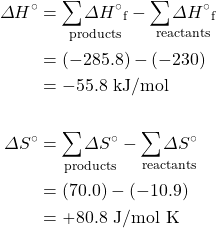The value of ΔG° at 298 K is: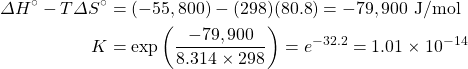1. Question and solution from Chem1 Virtual Textbook, Stephen Lower/CC-BY-SA-3.0
2. Question and solution from Chem1 Virtual Textbook, Stephen Lower/CC-BY-SA-3.0